Courses

# RD Sharma Solutions Ex-25.1, (Part - 1), Probability, Class 9, Maths Class 9 Notes | EduRev

## Class 9 : RD Sharma Solutions Ex-25.1, (Part - 1), Probability, Class 9, Maths Class 9 Notes | EduRev

The document RD Sharma Solutions Ex-25.1, (Part - 1), Probability, Class 9, Maths Class 9 Notes | EduRev is a part of the Class 9 Course RD Sharma Solutions for Class 9 Mathematics.
All you need of Class 9 at this link: Class 9

Q1. A coin is tossed 1000 times with the following sequence: Head: 455, Tail = :545. Compute the probability of each event

Answer: It is given that the coin is tossed 1000 times. The number of trials is 1000

Let us denote the event of getting head and of getting tails be E and F respectively. Then

Number of trials in which the E happens = 455

So, Probability of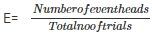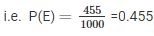Similarity, the probability of the event getting a tail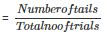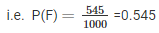Q2. Two coins are tossed simultaneously 500 times with the following frequencies of different outcomes:

Find the probability of occurrence of each of these events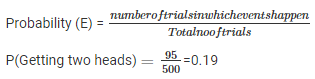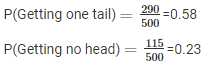Q3. Three coins are tossed simultaneously 100 times with the following frequencies of different outcomes:

If the three coins are tossed simultaneously again, compute the probability of:

3. At least one Head coming up

4. Getting more Tails than Heads

5. Getting more heads than tails

ANS:

1: Probability of 2 Heads coming up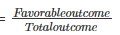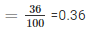2. Probability of 3 Heads coming up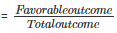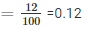3. Probability of at least one head coming up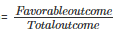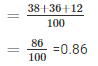4. Probability of getting more Heads than Tails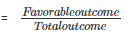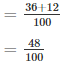5. Probability of getting more tails than heads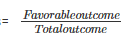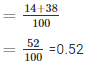Q4. 1500 families with 2 children were selected randomly, and the following data were recorded:

 No of girls in a family 0 1 2 No of girls 211 814 475

If a family is chosen at random, compute the probability that it has:

1. No girl

2. 1 girl

3. 2 girls

4. At most one girl

5. More girls than boys

1. Probability of having no girl in a family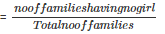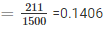2. Probability of having 1 girl in a family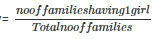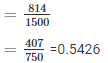3. Probability of having 2 girls in a family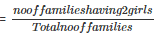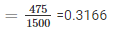4. Probability of having at the most one girl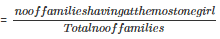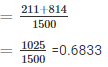5. Probability of having more girls than boys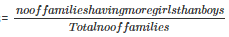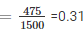Q5. In a cricket match, a batsman hits a boundary 6 times out of 30 balls he plays. Find the probability that:

1. He hit a boundary

2. He did not hit a boundary.

Number of times the batsman hits a boundary= 6

Total number of balls played= 30

Number of times the batsman did not hit a boundary= 30-6 = 24

1. Probability that the batsman hits a boundary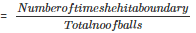= 6/30

= 1/5

2. Probability that the batsman does not hit a boundary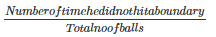= 24/30

= 4/5

Q6. The percentage of marks obtained by a student in the monthly unit tests are given below:

 UNIT TEST I II III IV V PERCENTAGE OF MARK OBTAINED 69 71 73 68 76

Find the probability that the student gets

1. More than 70% marks

2. Less than 70% marks

3. A distinction

1: Let E be the event of getting more than 70% marks

No of times E happens=3

Probability(Getting more than 70%)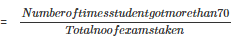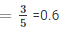2. Let F be the event of getting less than 70% marks

No of times F happen = 2

Probability(Getting less than 70%)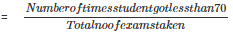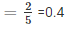3. Let G be the event of getting distinction

No of times G happen = 1

Probability(Getting distinction) =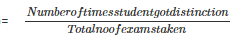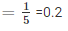Q7. To know the opinion of the students about Mathematics, a survey of 200 students were conducted. The data was recorded in the following table

 Opinion Like Dislike Number of students 135 65

Find the probability that student chosen at random:

1. Likes Mathematics

2. Does not like it.

1. Probability that a student likes mathematics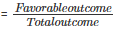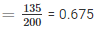2. Probability that a student does not like mathematics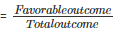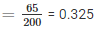Offer running on EduRev: Apply code STAYHOME200 to get INR 200 off on our premium plan EduRev Infinity!

91 docs

,

,

,

,

,

,

,

,

,

,

,

,

,

,

,

,

,

,

,

,

,

,

,

,

,

,

,

,

,

,

,

,

,

;